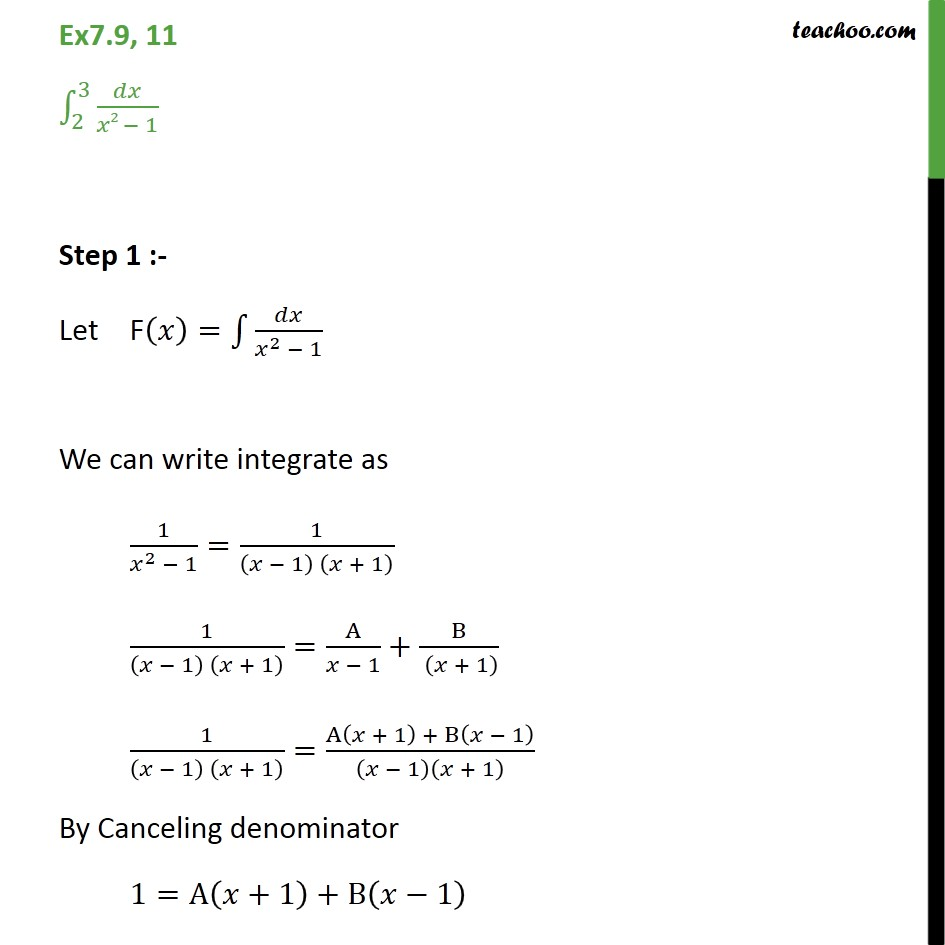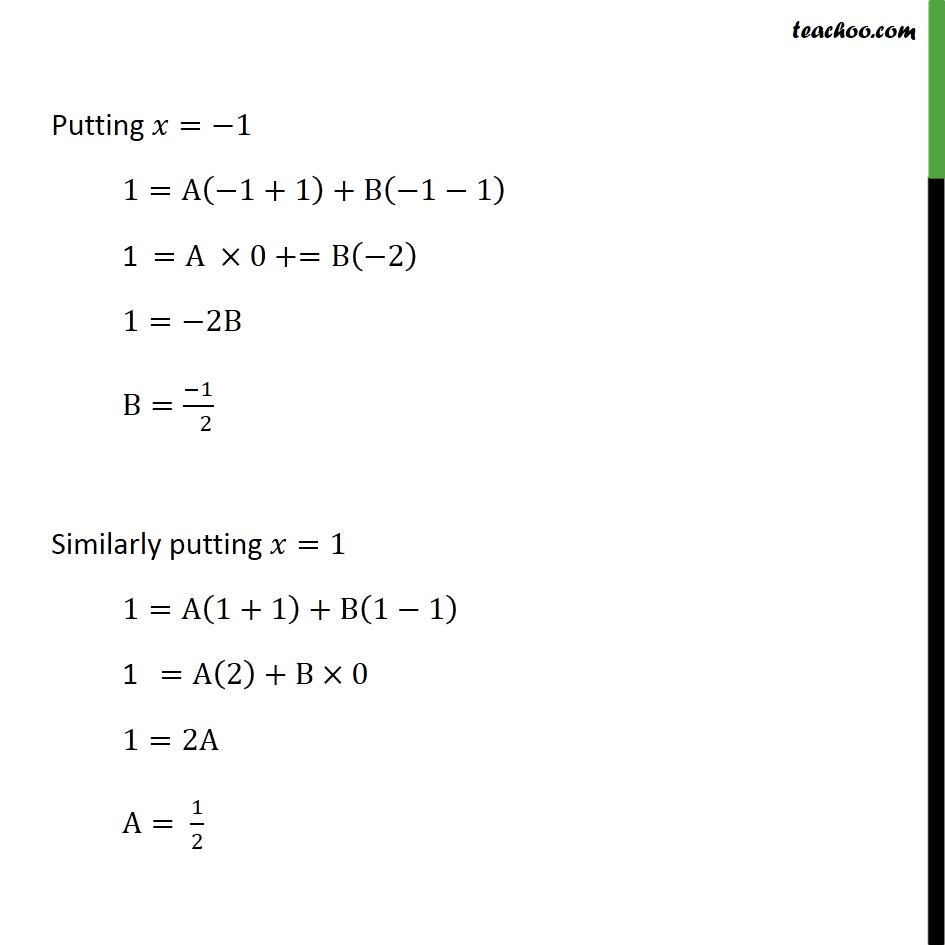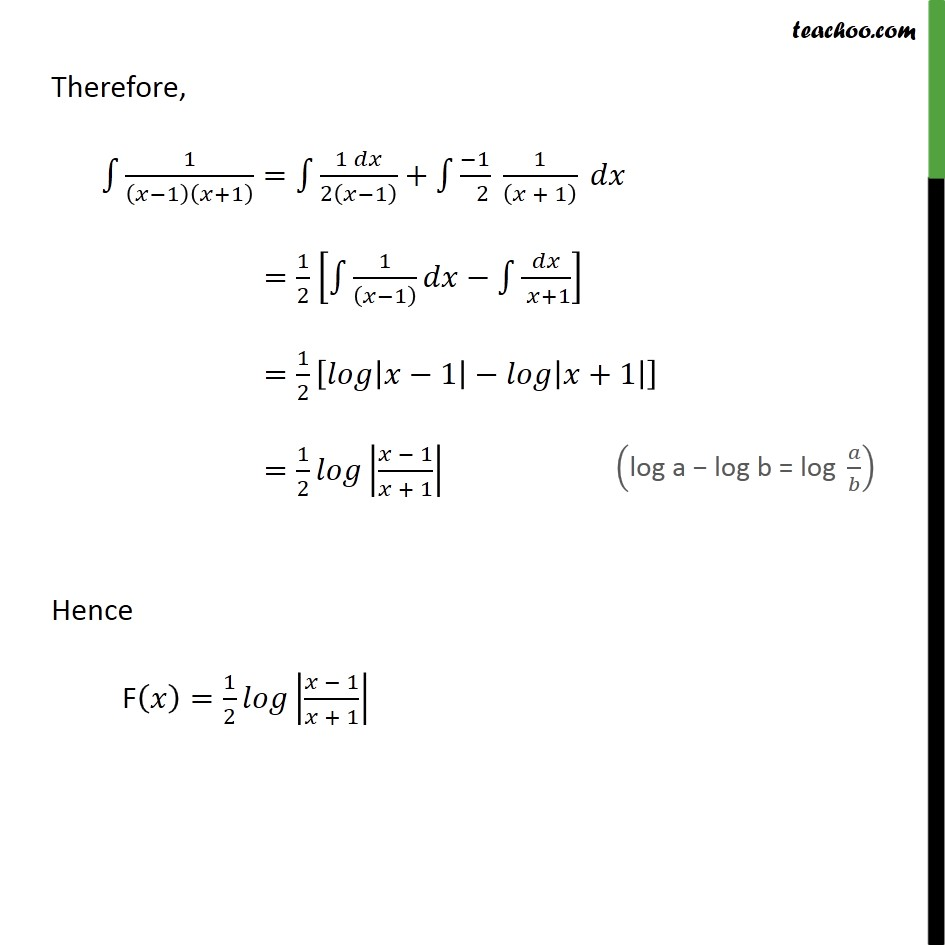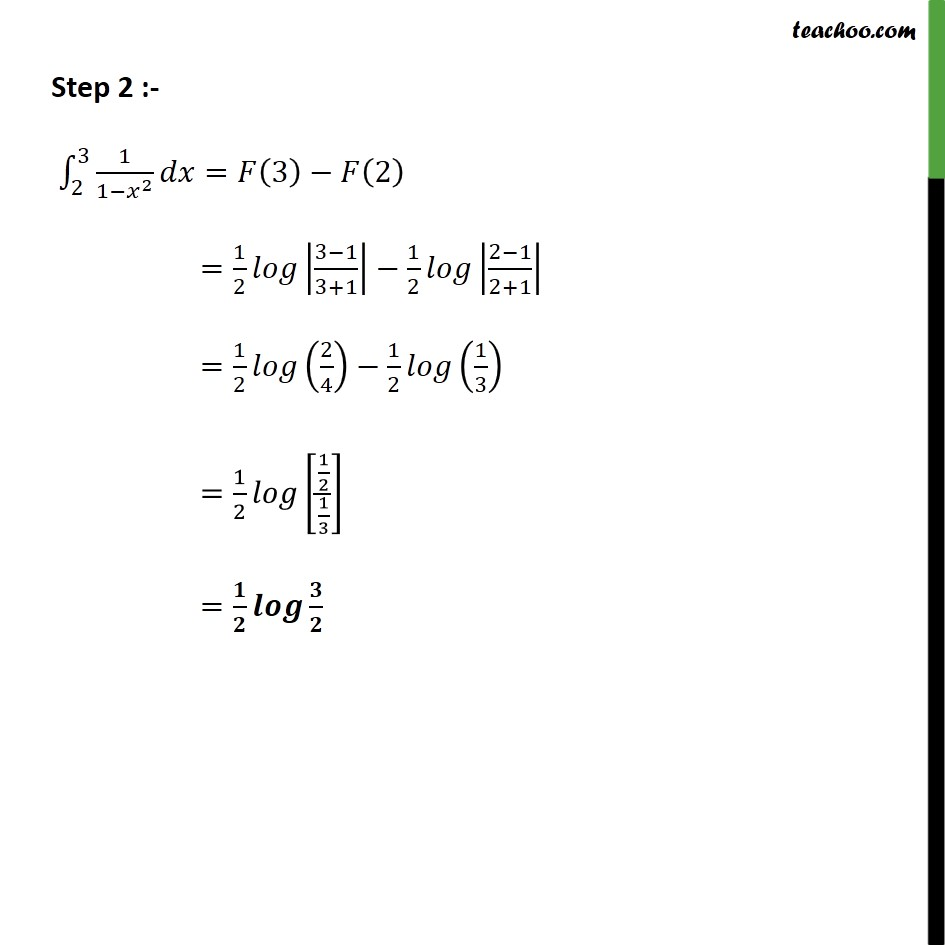Ex 7.8

Chapter 7 Class 12 Integrals
Serial order wiseLearn in your speed, with individual attention - Teachoo Maths 1-on-1 Class

### Transcript

Ex7.9, 11 2 3 2 1 Step 1 :- Let F = 2 1 We can write integrate as 1 2 1 = 1 1 + 1 1 1 + 1 = A 1 + B + 1 1 1 + 1 = A + 1 + B 1 1 + 1 By Canceling denominator 1=A +1 +B 1 Putting = 1 1=A 1+1 +B 1 1 1 =A 0+=B 2 1= 2B B= 1 2 Similarly putting =1 1=A 1+1 +B 1 1 1 =A 2 +B 0 1=2A A= 1 2 Therefore, 1 1 +1 = 1 2 1 + 1 2 1 + 1 = 1 2 1 1 +1 = 1 2 1 +1 = 1 2 1 + 1 Hence F = 1 2 1 + 1 Step 2 :- 2 3 1 1 2 = 3 2 = 1 2 3 1 3+1 1 2 2 1 2+1 = 1 2 2 4 1 2 1 3 = 1 2 1 2 1 3 =